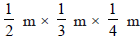Courses

# Mensuration - MCQ

## 10 Questions MCQ Test Class 8 Mathematics by VP Classes | Mensuration - MCQ

Description
This mock test of Mensuration - MCQ for Class 8 helps you for every Class 8 entrance exam. This contains 10 Multiple Choice Questions for Class 8 Mensuration - MCQ (mcq) to study with solutions a complete question bank. The solved questions answers in this Mensuration - MCQ quiz give you a good mix of easy questions and tough questions. Class 8 students definitely take this Mensuration - MCQ exercise for a better result in the exam. You can find other Mensuration - MCQ extra questions, long questions & short questions for Class 8 on EduRev as well by searching above.
QUESTION: 1

Solution:
QUESTION: 2

Solution:
QUESTION: 3

### If the parallel sides of a parallelogram are 2 cm apart and their sum is 10 cm then its area is:

Solution:

Distance between the two parallel lines = h =2 cm
sum of length of sides =10cm
As we know the sides of parallelogram are equal
let the length of side be 'b' =base of parallelogram
2b =10
b = 5 cm
area of parallelogram = h×b
= 2×5
=10 cm2

QUESTION: 4

Which of the following has its area and perimeter numerically equal?

Solution:
QUESTION: 5

If the edge of a cube is 1 cm then which of the following is its total surface area?

Solution:
QUESTION: 6

Which of the following is equal to 1 kilolitre?

Solution:
QUESTION: 7

If the dimensions of a room are l, b and h, (∴ l → length, l → breadth and h → hight) themwhich of the following is the area of its four walls?

Solution:
QUESTION: 8

If the dimensions of a room are 2 m, 3 and 4 m then which of the following is the number ofcubes of sizewhich can he placed is the room?

Solution:
QUESTION: 9

If base area of a room 12 m2 and height is 3 m then its volume is:

Solution:
QUESTION: 10

Two identical cubes each of total surface area of 6 cm2 are joined end to end. Which of thefollowing is the total surface area of the cuboid so formed?

Solution: